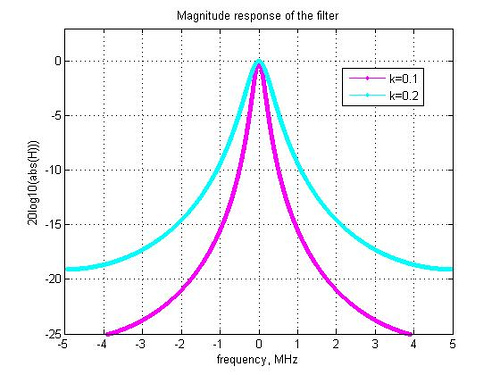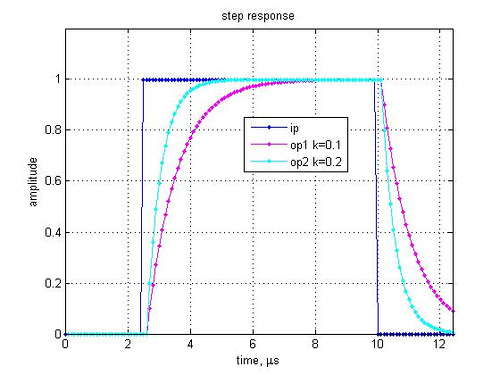(5 votes, average: 4.80 out of 5)Loading ...

Digital implementation of RC low pass filter

by on December 2, 2007

Thanks to the nice article from Xilinx TechXclusives [XLNX-TECH], let us try to understand the probable digital implementation of resistor-capacitor based low pass filter. Consider a simple RC filter shown in the figure below. Assuming that there is no load across the capacitor, the capacitor charges and discharges through the resistor path.Figure: RC low pass filter

is the voltage at the input of resistor and

is the voltage at the output.

From capacitor theory, the charge in the capacitor is , where

is the capacitance

is the voltage and

is the constant current flowing for short duration of time .

With the input voltage is greater than output voltage , resulting in current flowing through the resistor , where

.

When this current flows into the capacitor for a short time , the capacitor will charge and the voltage across the capacitor increases to

, where

is the new value of output voltage.

Digital Implementation

The above equation seems to be convenient for digital implementation as shown in the equation below:

where,

,

is ,

is and

is .

The transfer function of the above equation is

.

Simulation model

Script for plotting the frequency and step response of a digital RC low pass filter.Figure: Frequency response of the digital implementation of RC low pass filterFigure: Step response of the digital implementation of RC low pass filter

Observations

1. As expected, lower the value of k, tighter is the frequency response and slower is the settling time. This is in synch with analog RC implementation, where a higher value of R and C suggests that the capacitor takes more time to charge/discharge (Note that k is inversely proportional to RC).

2. To facilitate simpler digital implementations not involving multipliers, the value of k can be chosen to be a power of 2.

References

Hope this helps.

Krishna

D id you like this article? Make sure that you do not miss a new article by subscribing to RSS feed OR subscribing to e-mail newsletter. Note: Subscribing via e-mail entitles you to download the free e-Book on BER of BPSK/QPSK/16QAM/16PSK in AWGN.

Previous post:

Next post: